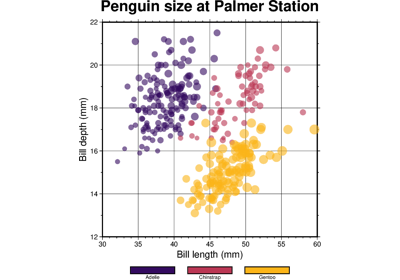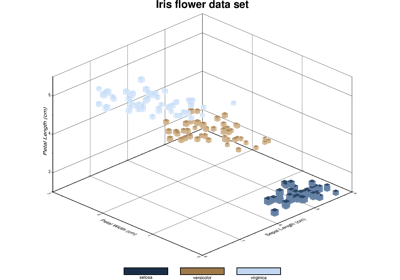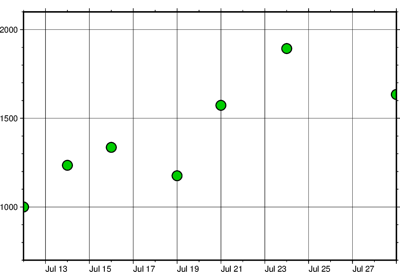# pygmt.info

pygmt.info(data, *, per_column=None, spacing=None, nearest_multiple=None, verbose=None, aspatial=None, coltypes=None, incols=None, registration=None, **kwargs)[source]

Reads from files and finds the extreme values in each of the columns reported as min/max pairs. It recognizes NaNs and will print warnings if the number of columns vary from record to record. As an option, it will find the extent of the first two columns rounded up and down to the nearest multiple of the supplied increments given by `spacing`. Such output will be in a numpy.ndarray form [w, e, s, n], which can be used directly as the `region` parameter for other modules (hence only dx and dy are needed). If the `per_column` parameter is combined with `spacing`, then the numpy.ndarray output will be rounded up/down for as many columns as there are increments provided in `spacing`. A similar parameter `nearest_multiple` will provide a numpy.ndarray in the form of [zmin, zmax, dz] for makecpt.

Full option list at https://docs.generic-mapping-tools.org/latest/gmtinfo.html

Aliases:

• C = per_column

• I = spacing

• T = nearest_multiple

• V = verbose

• a = aspatial

• f = coltypes

• i = incols

• r = registration

Parameters
• data (str or numpy.ndarray or pandas.DataFrame or xarray.Dataset or geopandas.GeoDataFrame) – Pass in either a file name to an ASCII data table, a 1-D/2-D `numpy.ndarray`, a `pandas.DataFrame`, an `xarray.Dataset` made up of 1-D `xarray.DataArray` data variables, or a `geopandas.GeoDataFrame` containing the tabular data.

• per_column (bool) – Report the min/max values per column in separate columns.

• spacing (str) – [b|p|f|s]dx[/dy[/dz…]]. Compute the min/max values of the first n columns to the nearest multiple of the provided increments [default is 2 columns]. By default, output results in the form `[w, e, s, n]`, unless `per_column` is set in which case we output each min and max value in separate output columns.

• nearest_multiple (str) – dz[+ccol]. Report the min/max of the first (0’th) column to the nearest multiple of dz and output this in the form `[zmin, zmax, dz]`.

• verbose (bool or str) –

Select verbosity level [Default is w], which modulates the messages written to stderr. Choose among 7 levels of verbosity:

• q - Quiet, not even fatal error messages are produced

• e - Error messages only

• w - Warnings [Default]

• t - Timings (report runtimes for time-intensive algorithms)

• i - Informational messages (same as `verbose=True`)

• c - Compatibility warnings

• d - Debugging messages

• aspatial (bool or str) – [col=]name[,…]. Control how aspatial data are handled during input and output. Full documentation is at https://docs.generic-mapping-tools.org/latest/gmt.html#aspatial-full.

• incols (str or 1-D array) –

Specify data columns for primary input in arbitrary order. Columns can be repeated and columns not listed will be skipped [Default reads all columns in order, starting with the first (i.e., column 0)].

• For 1-D array: specify individual columns in input order (e.g., `incols=[1,0]` for the 2nd column followed by the 1st column).

• For `str`: specify individual columns or column ranges in the format start[:inc]:stop, where inc defaults to 1 if not specified, with columns and/or column ranges separated by commas (e.g., `incols="0:2,4+l"` to input the first three columns followed by the log-transformed 5th column). To read from a given column until the end of the record, leave off stop when specifying the column range. To read trailing text, add the column t. Append the word number to t to ingest only a single word from the trailing text. Instead of specifying columns, use `incols="n"` to simply read numerical input and skip trailing text. Optionally, append one of the following modifiers to any column or column range to transform the input columns:

• +l to take the log10 of the input values.

• +d to divide the input values by the factor divisor [Default is 1].

• +s to multiple the input values by the factor scale [Default is 1].

• +o to add the given offset to the input values [Default is 0].

• coltypes (str) – [i|o]colinfo. Specify data types of input and/or output columns (time or geographical data). Full documentation is at https://docs.generic-mapping-tools.org/latest/gmt.html#f-full.

• registration (str) – g|p. Force gridline (g) or pixel (p) node registration [Default is g(ridline)].

Returns

output (np.ndarray or str) – Return type depends on whether any of the `per_column`, `spacing`, or `nearest_multiple` parameters are set.

## Examples using `pygmt.info`Color points by categories

Color points by categories3-D Scatter plots

3-D Scatter plotsPlotting datetime charts

Plotting datetime charts